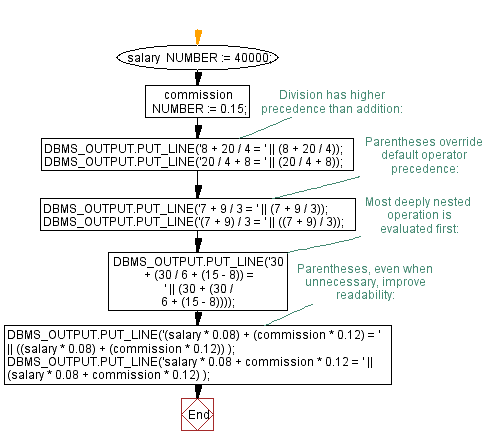﻿ PL/SQL Fundamentals Exercises: PL/SQL block to show the Operator Precedence - w3resource# PL/SQL Fundamentals Exercises: PL/SQL block to show the Operator Precedence

## PL/SQL Fundamentals: Exercise-11 with Solution

Write a PL/SQL block to show the operator precedence and parentheses in several more complex expressions.

PL/SQL Code:

``````DECLARE
salary      NUMBER := 40000;
commission  NUMBER := 0.15;
BEGIN
-- Division has higher precedence than addition:

DBMS_OUTPUT.PUT_LINE('8 + 20 / 4 = ' || (8 + 20 / 4));
DBMS_OUTPUT.PUT_LINE('20 / 4 + 8 = ' || (20 / 4 + 8));

-- Parentheses override default operator precedence:

DBMS_OUTPUT.PUT_LINE('7 + 9 / 3 = ' || (7 + 9 / 3));
DBMS_OUTPUT.PUT_LINE('(7 + 9) / 3 = ' || ((7 + 9) / 3));

-- Most deeply nested operation is evaluated first:

DBMS_OUTPUT.PUT_LINE('30 + (30 / 6 + (15 - 8)) = '
|| (30 + (30 / 6 + (15 - 8))));

-- Parentheses, even when unnecessary, improve readability:

DBMS_OUTPUT.PUT_LINE('(salary * 0.08) + (commission * 0.12) = '
|| ((salary * 0.08) + (commission * 0.12))
);

DBMS_OUTPUT.PUT_LINE('salary * 0.08 + commission * 0.12 = '
|| (salary * 0.08 + commission * 0.12)
);
END;
/
```
```

Sample Output:

```8 + 20 / 4 = 13
20 / 4 + 8 = 13
7 + 9 / 3 = 10
(7 + 9) / 3 = 5.33333333333333333333333333333333333333
30 + (30 / 6 + (15 - 8)) = 42
(salary * 0.08) + (commission * 0.12) = 3200.018
salary * 0.08 + commission * 0.12 = 3200.018

Statement processed.

0.01 seconds
```

Flowchart:Improve this sample solution and post your code through Disqus

What is the difficulty level of this exercise?

﻿